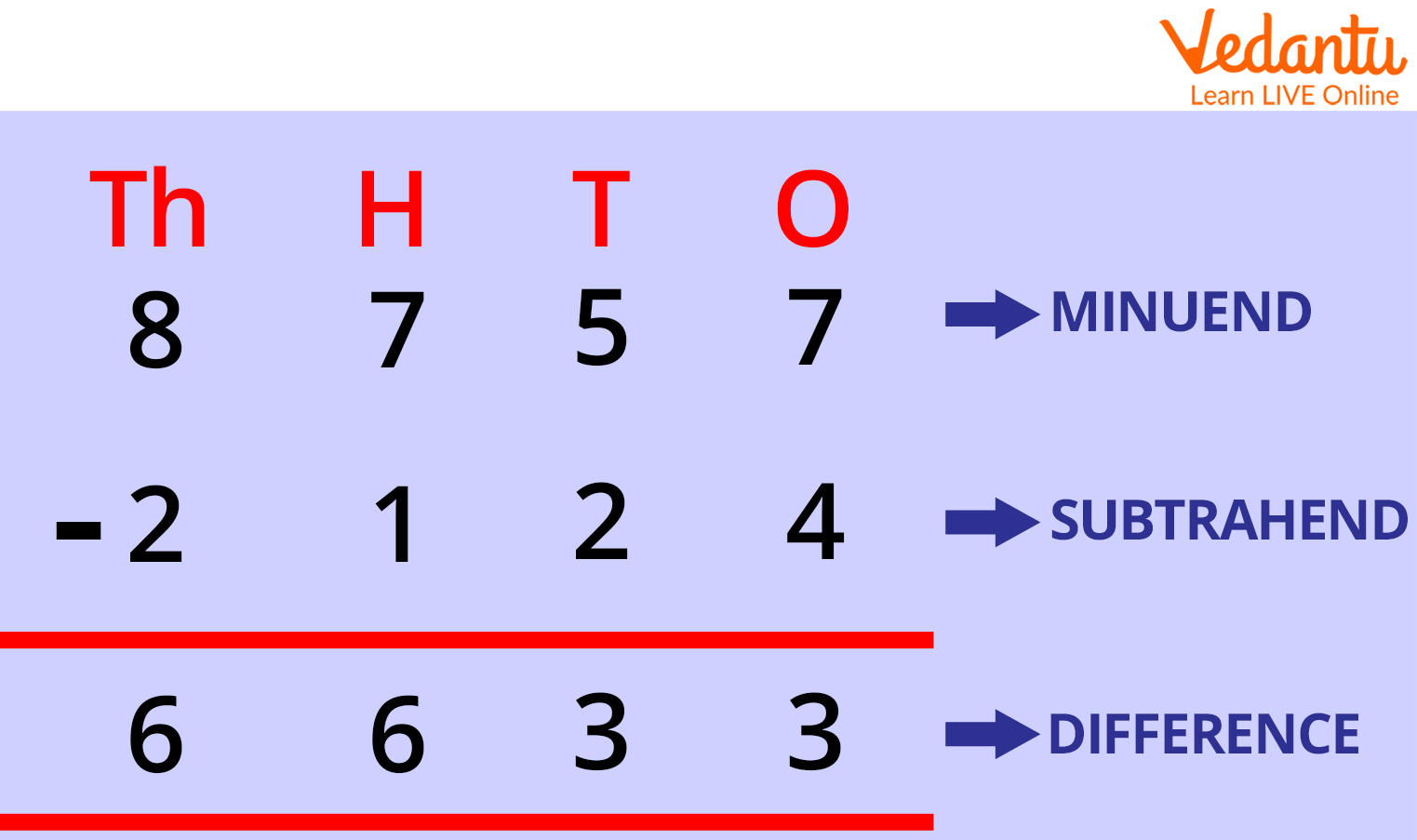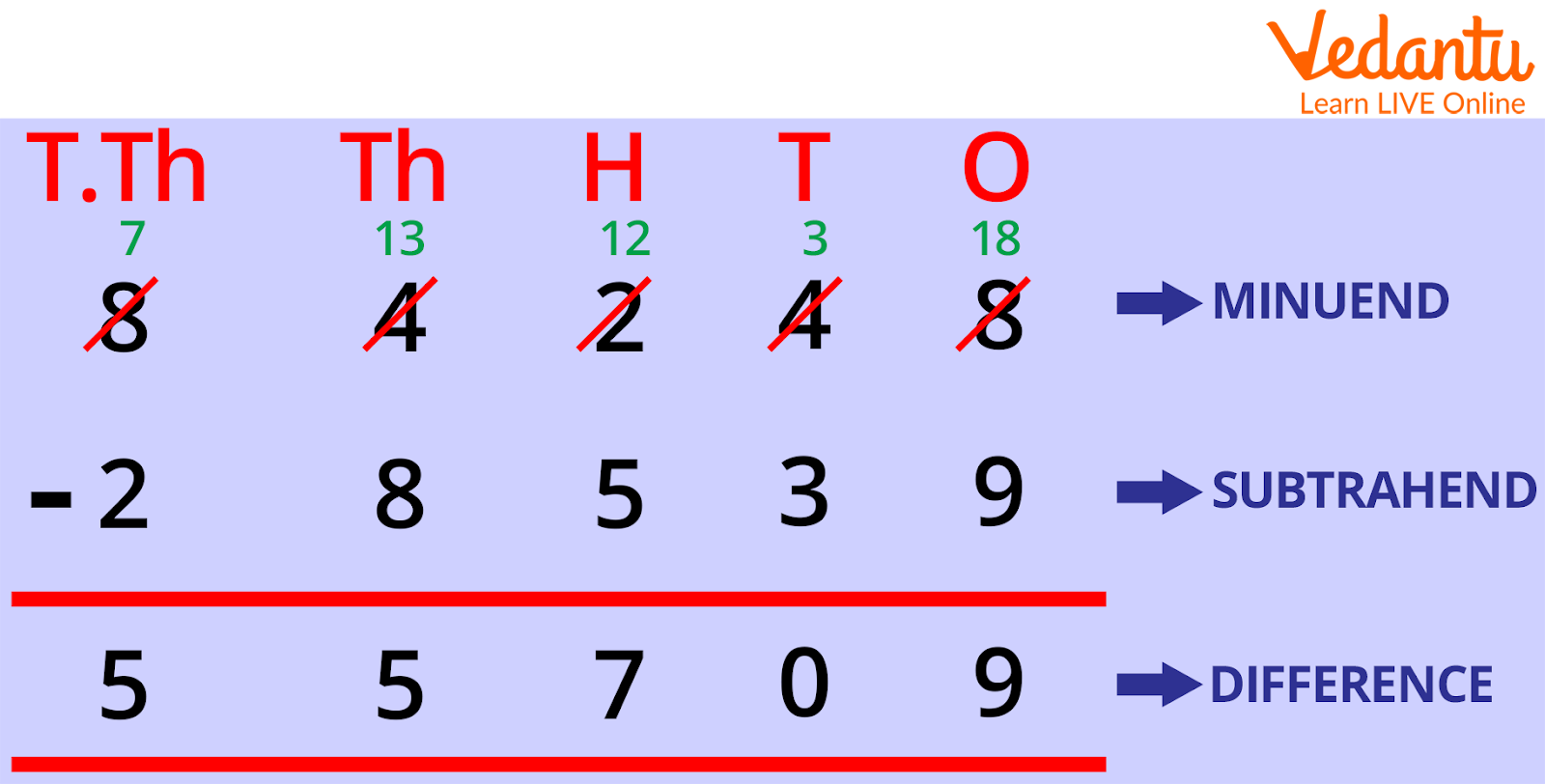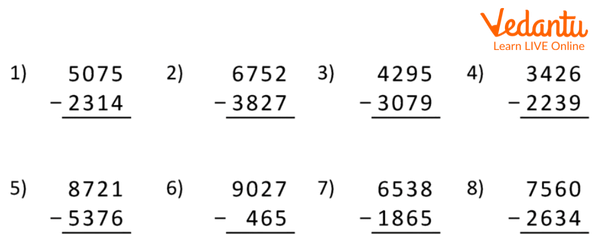Courses
Courses for Kids
Free study material
Free LIVE classes
More

# 4-Digit Subtraction with BorrowingLIVE
Join Vedantu’s FREE Mastercalss

## What is 4-Digit Subtraction?

When two integers are subtracted, the minuend must have a minimum of four digits and a maximum of three. This is known as 4-digit subtraction. When subtracting four digits, we do it one at a time in accordance with the place values of the digits—ones, tens, hundreds, and thousands.

A 4-digit number is subtracted from another 4-digit number in a 4-digit subtraction operation. You can deduct any smaller number from the 4-digit number as well. We all understand that the bigger number should go higher and the smaller one should go down. The subtrahend is the lower number inscribed below the minuend, which is the bigger number at the top.4-Digit Subtraction

## Steps for 4-Digit Subtraction

Step 1: First write the minuend on top and the subtrahend directly below to the minuend.

Step 2: The numbers must be placed as per their place values of ones, tens, hundreds, and thousands.

Step 3: Start subtracting the digits within the one’s column, followed by the tens column, the hundreds column, and therefore the thousands column.

Step 4: Once the difference is obtained, the corresponding answer is written underneath. This provides the difference between the two numbers.Subtraction

## 4-Digit Subtraction Without Regrouping

4-digit subtraction without regrouping means the numbers are subtracted with no borrowing. In such cases, all the digits within the minuend are bigger than the digits in the subtrahend. Let us understand this using an example.

Example: Subtract 2719 from 3989.

The 4-digit subtraction is often done using the following steps.

Step 1: Arrange the numbers consistent with their place value one below the other.

Step 2: Start subtracting the digits in one column. Since all the digits of the minuend are greater than the subtrahend, no regrouping/borrowing is required here. Subtract the numbers in one column and write the difference. (9 - 9 = 0)

Step 3: Then, subtract the numbers in the tens column and write the difference. (8 - 1 = 7).

Step 4: Then, subtract the numbers within the hundreds column and write the difference. (9 - 7 = 2).

Step 5: Now, subtract the numbers within the thousands column and write the difference. (3 - 2 = 1)

 3 9 8 9 - 2 7 1 9 1 2 7 0

Table Shows the Subtraction

## 4-Digit Subtraction With Regrouping

4-digit subtraction with regrouping is additionally known as 4-digit subtraction with borrowing. When any digit within the minuend (top row) is smaller than the corresponding digit in the subtrahend (bottom row), we regroup the numbers and then subtract. While regrouping, we borrow 1 number from the preceding column to form that particular digit in the minuend bigger than the corresponding digit of the subtrahend. Let us understand this with an example.

Example: Subtract 3678 from 8162.

Step 1: Arrange the numbers consistent with their place value. Start subtracting the digits in one column. We will see that 2 is smaller than 8. So, we'll borrow 1 from the tens column which will make it 12. Now, 12 - 8 = 4.

Step 2: After giving 1 to that column in the previous step, 6 becomes 5. Now, allow us to subtract the digits in the tens place (5 - 7). Here, 5 is smaller than 7. So, we'll borrow 1 from the hundreds column. This may make it 15. So, 15 - 7 = 8.

Step 3: In step 2 we had given 1 to the tens column, so we've 0 in the hundreds place. Here, 0 is smaller than 6, so we'll borrow 1 from the thousands column. This may make it 10. So, 10 - 6 = 4.

Step 4: Now, allow us to subtract the digits in the thousands place. After giving 1 to the hundreds column, 8 becomes 7. So, 7 - 3 = 4.

 8 1 6 2 - 3 6 7 8 4 4 8 4

Table Shows the Subtraction

Therefore, the difference between the 2 given numbers is 8162 - 3678 = 4484.

## Important Notes on 4-Digit Subtraction

• Always remember to rearrange the 4-digit number as per the place value of the digits.

• In the case of regrouping/borrowing, as and once we borrow, we subtract the numbers simultaneously.

Or

## Math Subtraction WorksheetsFour Digit Subtraction Worksheets with Pictures

Solutions:

1)

 5 0 7 5 - 2 3 1 4 2 7 6 1

2)

 6 7 5 2 - 3 8 2 7 2 9 2 5

3)

 4 2 9 5 - 2 2 3 9 2 0 5 6

4)

 3 4 2 6 - 2 2 3 9 1 1 8 7

5)

 8 7 2 1 - 5 3 7 6 3 3 4 5

6)

 9 0 2 7 - 4 6 5 8 5 6 2

7)

 6 5 3 8 - 1 8 6 5 4 6 7 3

8)

 7 5 6 0 - 2 6 3 4 4 9 2 6

## Summary

We have covered what a four-digit number is and how we can subtract a four-digit number. Never forget to enter the four numerals in the correct order according to their place value. When regrouping or borrowing, we concurrently subtract the numbers as we borrow. We have also solved one worksheet to understand it better. Solving questions always makes us understand better.

Last updated date: 01st Oct 2023
Total views: 67.5k
Views today: 0.67k

## FAQs on 4-Digit Subtraction with Borrowing

1. What is the difference between a four-digit and three-digit number subtraction?

4-digit subtraction is the difference between a 4-digit number and another 4-digit number. and the 3-digit number is the difference between only three-digit numbers.

2. Define the term borrowing?

Borrowing the process of taking an extra number obtained from any arithmetic operation to the other number.

3. Can we subtract a 3-digit number from a 4-digit number?

Yes, we can subtract.